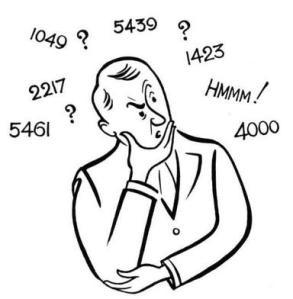# Mathematical Relationship aptitude puzzle

505.6K Views

Find the number to replace the question mark to complete the series, provided the pair of numbers exhibits a similar relationship?

? : 4623 :: 9 : 647Explanation –
The relationship holds for below formula :
pow(x,3) – pow(x,2) – 1
= 9*9*9 – 9*9 – 1
= 729 – 81 – 1
= 647

Similarly,
17*17*17 – 17*17 – 1
= 4913 – 289 – 1
= 4623